How to Add, Subtract, Multiply, and Divide Fractions

Introduction

Before you can go on to master more advanced concepts in algebra and geometry, you need to first master all mathematical functions relating to fractions. In this article, we will review how to add, subtract, multiply, and divide two fractions as well as a fraction and an integer. We'll also introduce complex fractions along with methods for simplifying them. Before your proceed though, make sure you fully understand the four basic mathematical operations: adding, subtracting, multiplying and dividing.

Key Terms

o Common denominator

o Reciprocal

o Complex fraction

Objectives

o Learn

o Understand how to interpret fractions that involve negative numbers

o Recognize and simplify complex fractions

Now that we have developed a solid foundation regarding what fractions are as well as some different types of fractions, we can now turn to application of the basic arithmetic operations (addition, subtraction, multiplication, and division) to fractions.

In cases that involve simple numbers, addition and subtraction of fractions is easy enough. For instance, adding one third and one third obviously gives us two thirds. Likewise, three fifths minus two fifths is one fifth. The first case is illustrated below.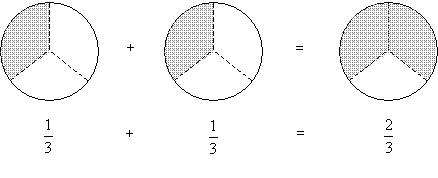But what about cases such as one half plus one third?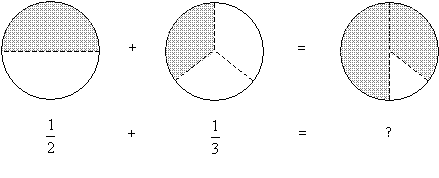Notice that adding (subtracting) fractions with the same denominator is very simple-we simply add (subtract) the numerators and divide by the same denominator. We should already know that we can write equivalent fractions that have different numerators and denominators. Thus, if we simply convert one or both of the fractions that we are adding or subtracting into equivalent fractions with the same denominator, then we are able to add the fractions in the simple manner described above. Then, if necessary, we can reduce the result to lowest terms.

The challenge in adding and subtracting fractions is finding a common denominator. The most straightforward approach to finding a common denominator is to simply multiply the two existing denominators and then convert the numerators accordingly to create equivalent fractions. Although this approach is conceptually simple, it can be mathematically difficult when the denominators are large. Nevertheless, let's try this approach for the purpose of illustration. Consider the addition mentioned above.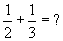A common denominator is 6 (or 2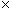3), because we can multiply the numerator and denominator of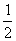by 3 to get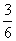, and we can multiply the numerator and denominator of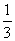by 2 to get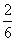. The addition is then straightforward.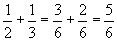Practice Problem: Calculate the result in each case.

a.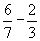b.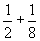c.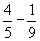Solution: In each case, find a common denominator and convert the terms to equivalent fractions with that denominator. One possible common denominator is given for each case. The sum (difference) of the fractions is the sum (difference) of the numerators over the common denominator. Reduce the result to lowest terms if applicable.

a. Common denominator: 21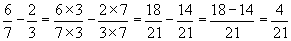b. Common denominator: 8

Interested in learning more? Why not take an online Pre-Algebra course?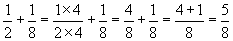c. Common denominator: 45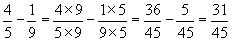Multiplication and Division

Multiplying and dividing fractions is in some ways simpler than adding and subtracting them. Let's say we want to multiplyby. Intuitively, the answer is fairly obvious: half of a half is a quarter (or one-fourth--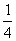). For instance, if you have 50 cents (half of a dollar) and you want to multiply it by a half, then you end up with 25 cents (a quarter of a dollar).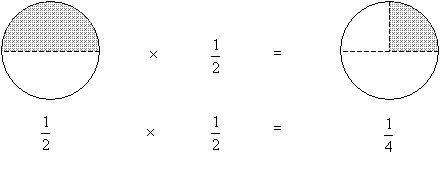To multiply two fractions, then, simply multiply the numerators and multiply the denominators to get the product. In some cases, the product will already be in lowest terms; in others, you may need to reduce it to lowest terms. For instance, the product of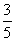and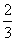is the following: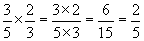When multiplying a fraction by an integer, note that any integer is simply a fraction with the integer as the numerator and 1 as the denominator. For instance,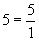Practice Problem: Calculate the following products.

a.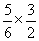b.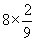c.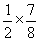Solution: In each case, the product is the product of the numerators over the product of the denominators. If one of the factors is an integer, treat it as a fraction having the integer as its numerator and 1 as its denominator. Reduce the product to lowest terms if applicable.

a.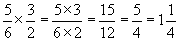b.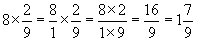c.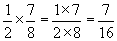Now consider the case of division. Let's say we want to divideby. Intuitively, the answer is 2--for instance, 25 cents (a quarter dollar) can go into 50 cents (a half dollar) twice.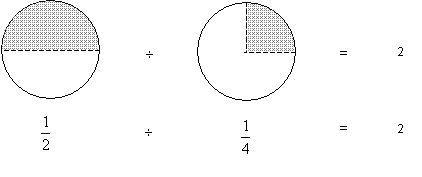Notice above that if we were to flip the second factor so that the numerator became the denominator and the denominator became the numerator and also changed the operation from division to multiplication, we would end up with the same result.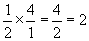This is, in fact, a convenient way to divide fractions. Division by a fraction is the same as multiplication by the reciprocal of that fraction. A reciprocal is simply a "flipped" fraction. Thus, for instance, the reciprocal of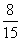is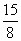(or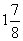).

As with multiplication of fractions, remember that an integer can also be written as a fraction. Thus, for instance, the reciprocal of 6 is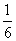. We can therefore divide fractions by integers as well as by other fractions. In addition, note that the product of a fraction and its reciprocal is always 1. Consider the example below.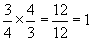In light of how we have defined division and multiplication, we can provide a more rigorous justification of our method for calculating equivalent fractions. Note that the number 1 can be written as any other number divided by itself. For instance,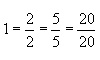Thus, the process of finding equivalent fractions is nothing more than multiplying a given fraction by 1! Consider the example below.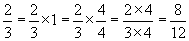Practice Problem: Calculate the following quotients.

a.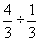b.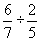c.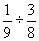Solution: In each case, multiply the dividend by the reciprocal of the divisor. Reduce the product to lowest terms if applicable.

a.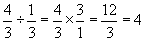b.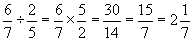c.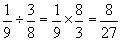Fractions and Negative Numbers

Because fractions are nothing more than a representation of division, we already have the tools we need to understand the role of negative numbers in fractions. Recall that the product (or quotient) of two negative or two positive numbers is positive and that the product (or quotient) of one negative number and one positive number is negative. Thus, consider the example of the fraction; we'll consider each possible case.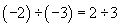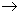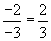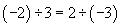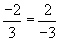In the first case (both numerator and denominator have the same sign), the result is a positive number. In the second case (numerator and denominator have opposite signs), the result is a negative number. Thus, we may sometimes simply place the negative sign in the second case next to the entire fraction rather than next to the numerator or denominator. Nevertheless, note that all three representations are equal, and in some situations, one may be more useful than another.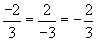Complex Fractions

Recall that a fraction is simply a way of expressing division of two numbers (where the numerator is the dividend and the denominator is the divisor). Since we can divide fractions, we can also express this division as a "fraction of fractions," or a complex fraction. An example of a complex fraction is given below. Note that for clarity, the fractions in the numerator and denominator of the complex fraction are shown "slanted"--this change does not imply any mathematical difference, however.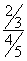Such fractions can and often should be simplified. To do so, we can take one of several approaches. Recall that we can find an equivalent fraction by multiplying the numerator and denominator by the same number. Thus, one approach is to multiply both numerator and denominator of the complex fraction by the product of the simple fractions' denominators, as shown below.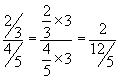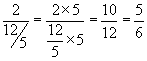Alternatively, we can multiply both numerator and denominator of the complex fraction by the reciprocal of its denominator. Because the denominator then becomes 1, the result is simply the value of the numerator.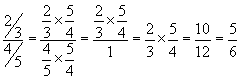Another way of looking at this last approach is that we are simply performing the division: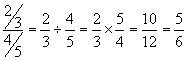Depending on the particular situation, one approach may be simpler than another; all are equally acceptable, however.

Practice Problem: Simplify the following complex fractions.

a.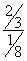b.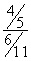c.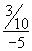Solution: One possible approach to simplifying these complex fractions is to multiply the fraction in the numerator by the reciprocal of the fraction in the denominator. Reduce the result to lowest terms if applicable. In the case of part c, note that the reciprocal of 5 is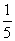and that the quotient (or product) of a positive number divided (multiplied) by a negative number is a negative number.

a.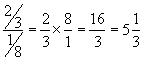b.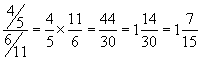c.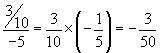If you are still having problems with fractions, you may also want to check out this article here: How to do Fractions the Easy Way.